# 蒙哥马利算法详解

• 蒙哥马利乘模，是用来计算xy (mod N)$x\cdot y\ (mod\ N)$
• 蒙哥马利约减，是用来计算tρ1 (mod N)$t\cdot \rho^{-1}\ (mod\ N)$
• 蒙哥马利幂模，是用来计算xy (mod N)$x^y\ (mod\ N)$

### 基本概念

ZN={0,1,2,,N1}$Z_N=\left\{0,1,2,\cdots,N-1\right\}$

1. 正整数
2. 最大长度是lN$l_N$

0x+y2(N1)$\qquad 0\leqslant x+y\leqslant 2\cdot(N-1)$

(N1)xy(N1)$-(N-1)\leqslant x-y\leqslant (N-1)$

0xy(N1)2$0\leqslant x\cdot y\leqslant (N-1)^2$

tNtN$t-（N\cdot \lfloor\frac{t}{N}\rfloor）$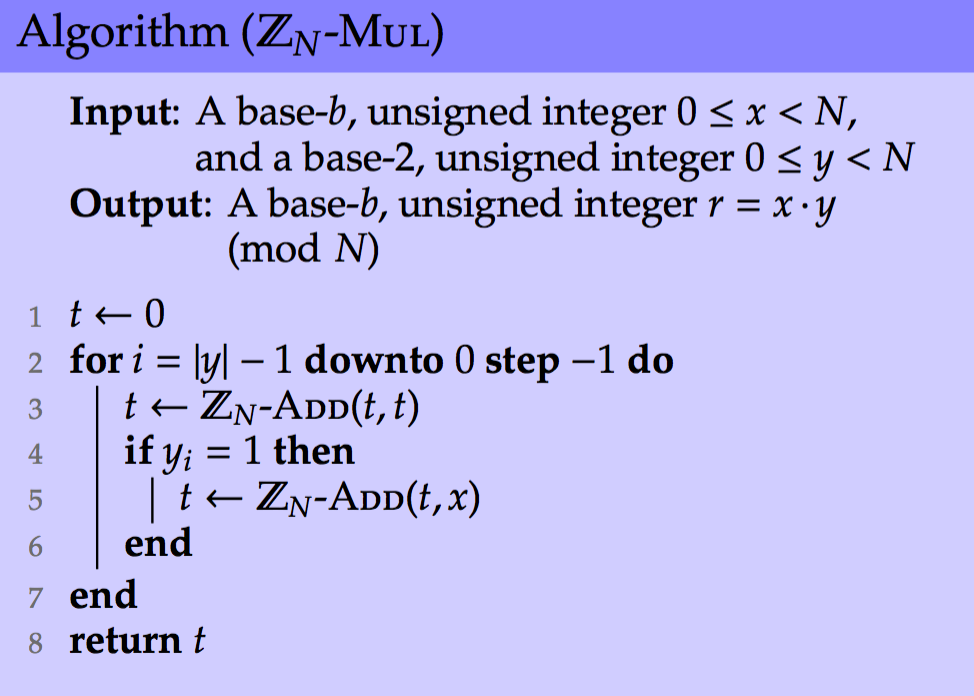1，根据以下公式，来计算取模操作

tNtN$t-（N\cdot \lfloor\frac{t}{N}\rfloor）$

• 整个计算过程是基于标准的数字表示
• 不需要预计算（也就是提前计算一些变量，以备使用）
• 涉及到一个除法操作，非常费时和复杂

2，用Barrett reduction算法，这篇文章不细说，但是有以下特征

• 基于标准的数字表示
• 不需要预计算
• 需要2(lN+1)(lN+1)$2 \cdot (l_N+1) \cdot (l_N+1)$ 次数乘运算

3，用蒙哥马利约减，也就是下面要讲的算法，有以下特征

• 不是基于标准的数字表示（后文中有提到，是基于蒙哥马利表示法）
• 需要预计算
• 需要2(lN)(lN)$2 \cdot (l_N) \cdot (l_N)$ 次数乘运算

### 蒙哥马利预备知识

xy=xsumly1i=0yibi$x\cdot y=x\cdot sum_{i=0}^{l_y-1}y_i \cdot b^i$

=sumly1i=0yixbi$\qquad=sum_{i=0}^{l_y-1}y_i \cdot x \cdot b^i$

xy=(y0x100)+(y1x101)+(y2x102)$x\cdot y=(y_{0}\cdot x\cdot 10^{0})+(y_{1}\cdot x\cdot 10^{1})+(y_{2}\cdot x\cdot 10^{2})$
=(y0x0)+(y1x10)+(y2x100)$\qquad=(y_{0}\cdot x\cdot 0)+(y_{1}\cdot x\cdot 10)+(y_{2}\cdot x\cdot 100)$
=(y0x)+10(y1x+10(y2x+10(0)))$\qquad=(y_{0}\cdot x)+10\cdot(y_{1}\cdot x+10\cdot(y_{2}\cdot x\cdot +10\cdot(0)))$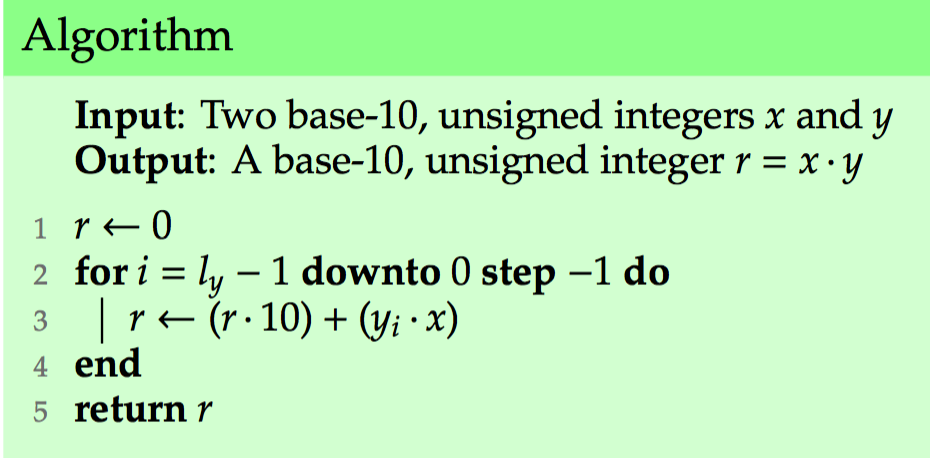xy=(y_0x)+10(y_1x+10(y_2x+10(0)))$x\cdot y=(y\_{0}\cdot x)+10\cdot(y\_{1}\cdot x+10\cdot(y\_{2}\cdot x\cdot +10\cdot(0)))$

xy1000=(y0x100)+(y1x101)+(y2x102)1000$\frac{x\cdot y}{1000}=\frac{(y_{0}\cdot x\cdot 10^{0})+(y_{1}\cdot x\cdot 10^{1})+(y_{2}\cdot x\cdot 10^{2})}{1000}$

=(y0x0)+(y1x10)+(y2x100)1000$\qquad=\frac{(y_{0}\cdot x\cdot 0)+(y_{1}\cdot x\cdot 10)+(y_{2}\cdot x\cdot 100)}{1000}$

=(y0x)1000+(y1x)100+(y2x)10$\qquad=\frac{(y_{0}\cdot x)}{1000}+\frac{(y_{1}\cdot x)}{100}+\frac{(y_{2}\cdot x)}{10}$

=(((((y0x)/10)+y1x)/10)+y2x)/10$\qquad=(((((y_0\cdot x)/10)+y_1\cdot x)/10)+y_2\cdot x)/10$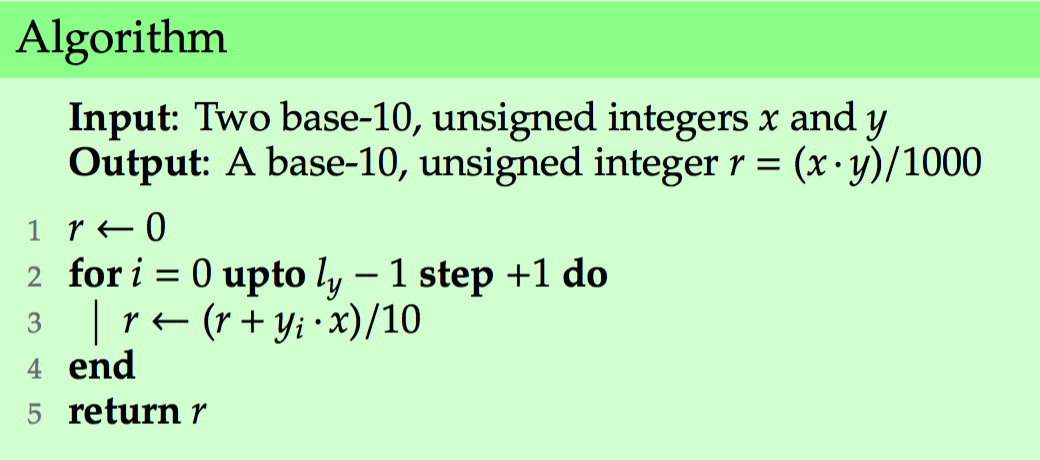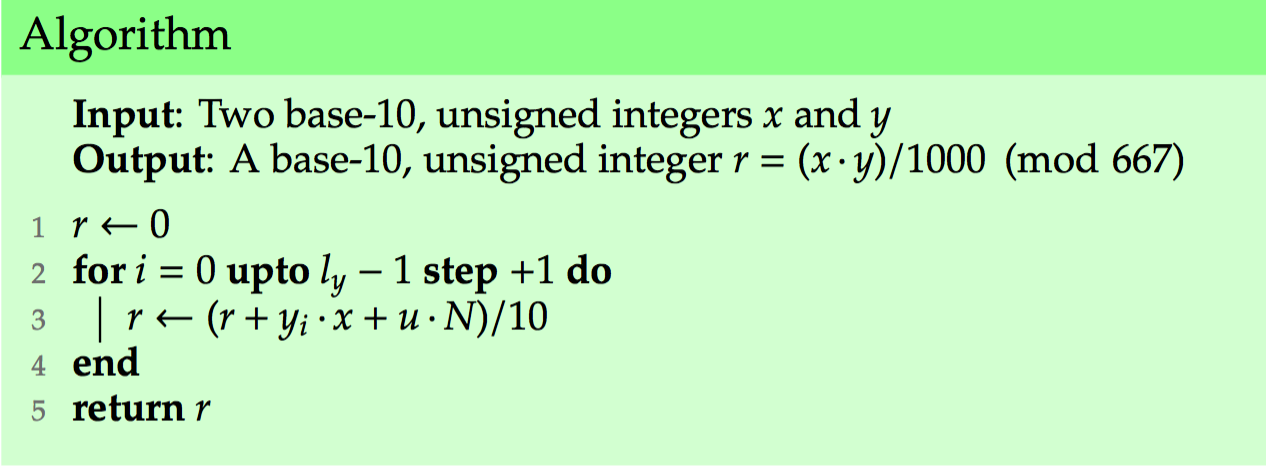- 第一个是输入x和y，计算xyρ1$x \cdot y \cdot \rho^{-1}$
- 第二个算法，输入一个t，计算tρ1$t \cdot \rho^{-1}$

xy (mod 667)=((x1000)(y1000)/1000)/1000 (mod 667)$x\cdot y\ (mod\ 667)=((x\cdot1000)\cdot(y\cdot1000)/1000)/1000\ (mod\ 667)$

• 蒙哥马利参数
给定一个N，N在b进制（例如，二进制时，b=2）下共有l位，gcd(N,b)=1$gcd(N,b)=1$，先预计算以下几个值(这就是前面提到的特性之一，需要预计算）：
• ρ=bk$\rho = b^k$ 指定一个最小的k，使得bk>N$b^k>N$
• ω=N1(mod ρ)$\omega = -N^{-1} (mod\ \rho)$
这两个参数是做什么用的呢，你对照前面的演变过程可以猜到ρ$\rho$ 就是前面演变中的1000，而ω$\omega$ 则是用来计算前面提到的u的。
• 蒙哥马利表示法
对于x，0xN1$0\leqslant x\leqslant N-1$，x的蒙哥马利表示法表示为x=xρ (mod N)$x=x\cdot \rho\ (mod\ N)$

### 蒙哥马利约减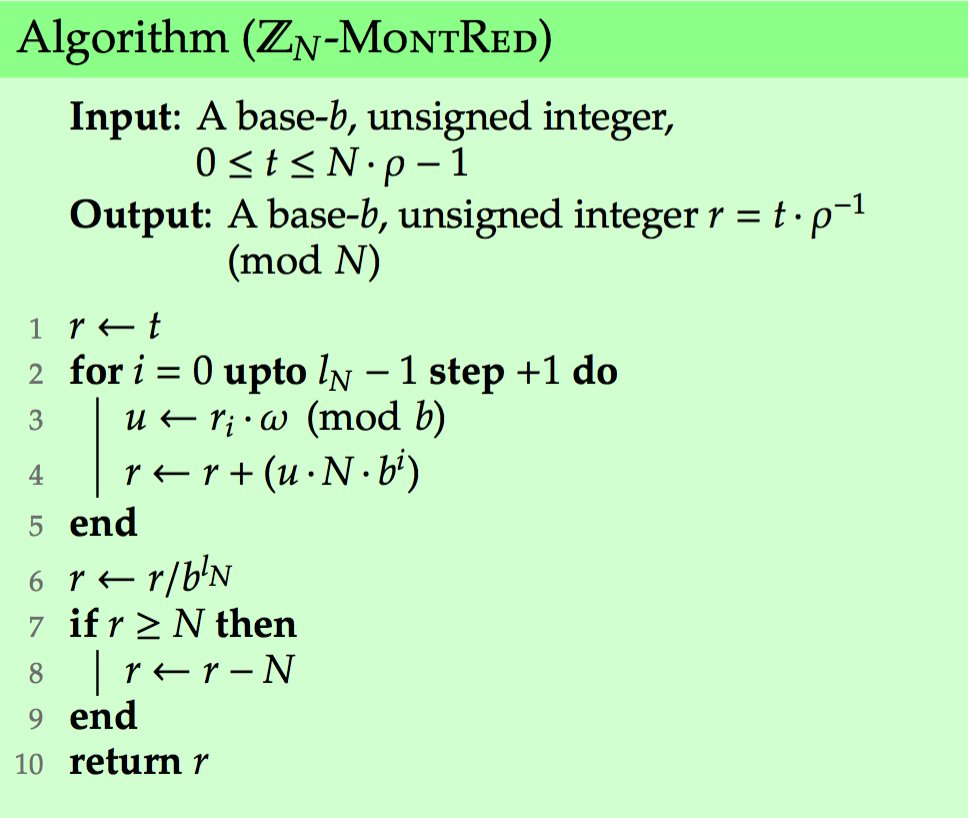### 蒙哥马利乘模

x̂ =xρ (mod N)$\hat{x}=x\cdot\rho\ (mod\ N)$
ŷ =yρ (mod N)$\hat{y}=y\cdot\rho\ (mod\ N)$

t=x̂ ŷ $t=\hat{x}\cdot\hat{y}$
=(xρ)(yρ)$\ =(x\cdot\rho)\cdot(y\cdot\rho)$
=(xy)ρ2$\ =(x\cdot y)\cdot\rho^2$

t̂ =(xy)ρ (mod N)$\hat{t}=(x \cdot y) \cdot \rho\ (mod\ N)$

b=10，也就是说在10进制下，N=667
bk>N$b^k>N$的最小的k是3，所以ρ=bk=103=1000$\rho=b^k=10^3=1000$
ω=N1 (mod ρ)=6671 (mod ρ)=997$\omega=-N^{-1}\ (mod\ \rho)=-667^{-1}\ (mod\ \rho)=997$

x̂ ŷ ρ1=(42110004221000)10001 (mod 667)$\hat{x}\cdot\hat{y}\cdot\rho^{-1}=(421\cdot1000\cdot422\cdot1000)\cdot1000^{-1}\ (mod\ 667)$
(421422)1000 (mod 667)$\qquad\qquad(421\cdot422)\cdot1000\ (mod\ 667)$
(240)1000 (mod 667)$\qquad\qquad(240)\cdot1000\ (mod\ 667)$
547 (mod 667)$\qquad\qquad547\ (mod\ 667)$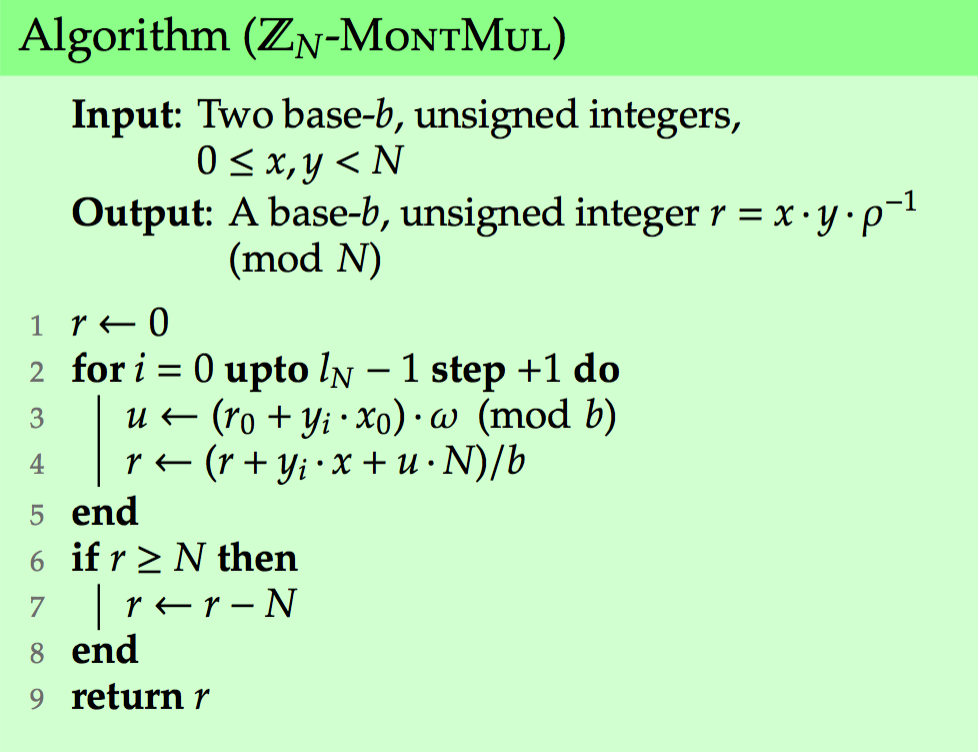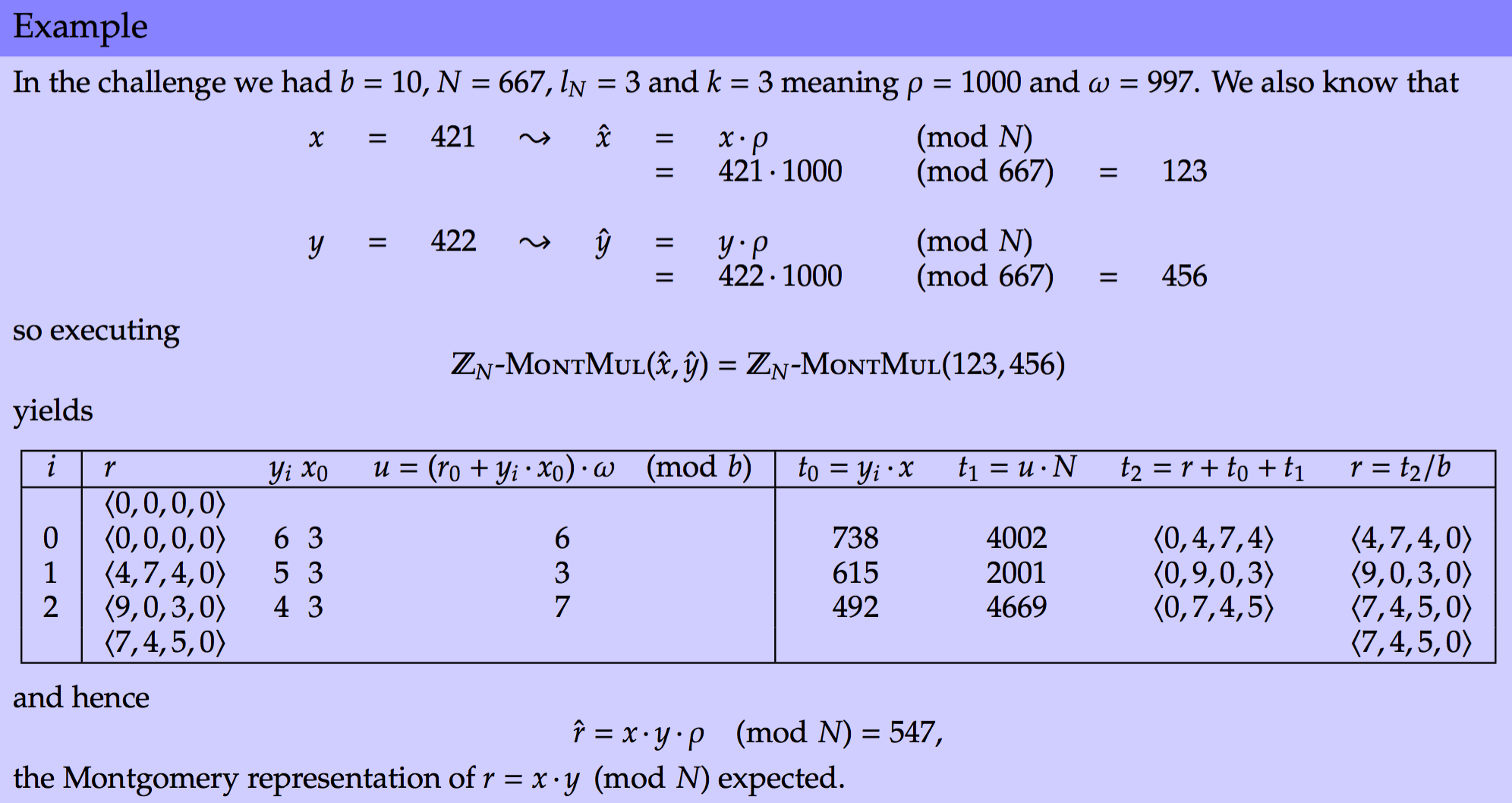### 蒙哥马利幂模

C1 =C*C % N =C^2 % N
C2 =C1*C % N =C^3 % N
C3 =C2*C2 % N =C^6 % N
C4 =C3*C % N =C^7 % N
C5 =C4*C4 % N =C^14 % N
C6 =C5*C % N =C^15 % N

D=1
WHILE E>0
IF E%2=0
C=C*C % N
E=E/2
ELSE
D=D*C % N
E=E-1
RETURN D

D=1
FOR i=n TO 0
D=D*D % N
IF E=1
D=D*C % N
RETURN D这样，模幂运算就转化成了一系列的模乘运算。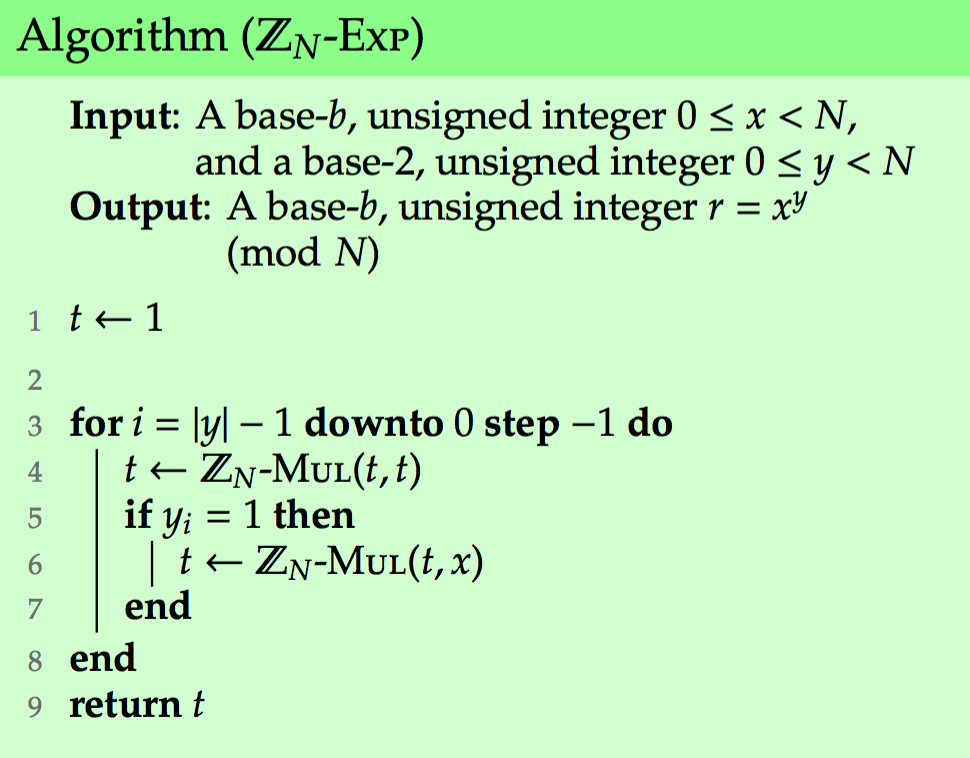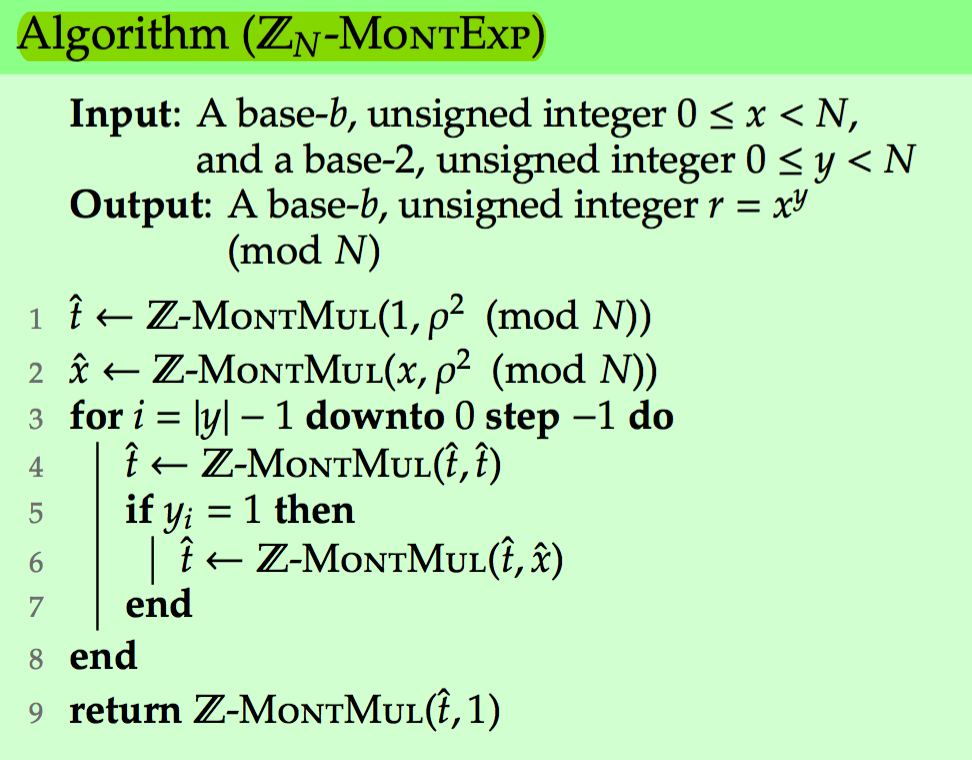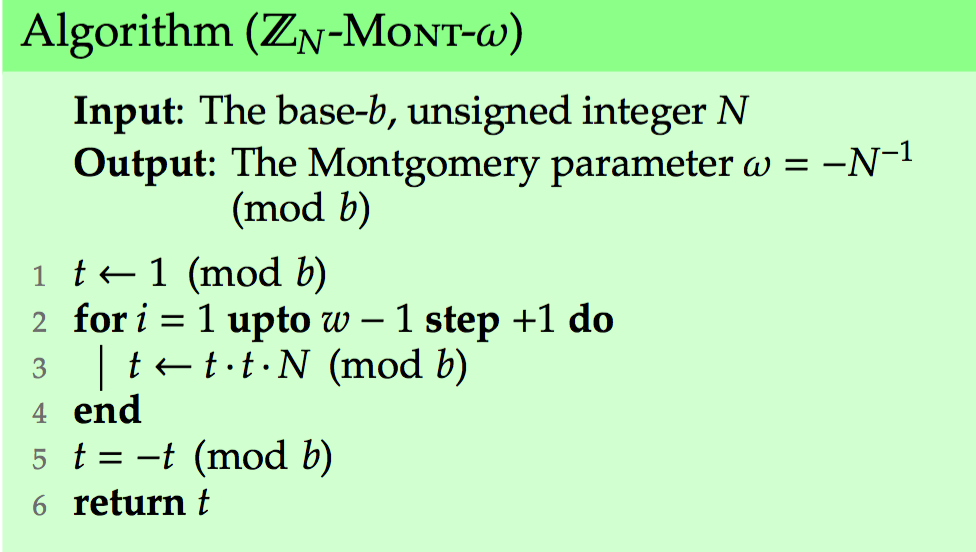b=2ω$b=2^{\omega}$ 所以ρ=bk=(2ω)k$\rho=b^k=({2^{\omega}})^k$

ρ2=(2w)k)2=22kω=22lNω$\rho^2={({2^w})^k)}^2=2^{2\cdot k\cdot \omega}=2^{2\cdot l_N\cdot \omega}$，算法如下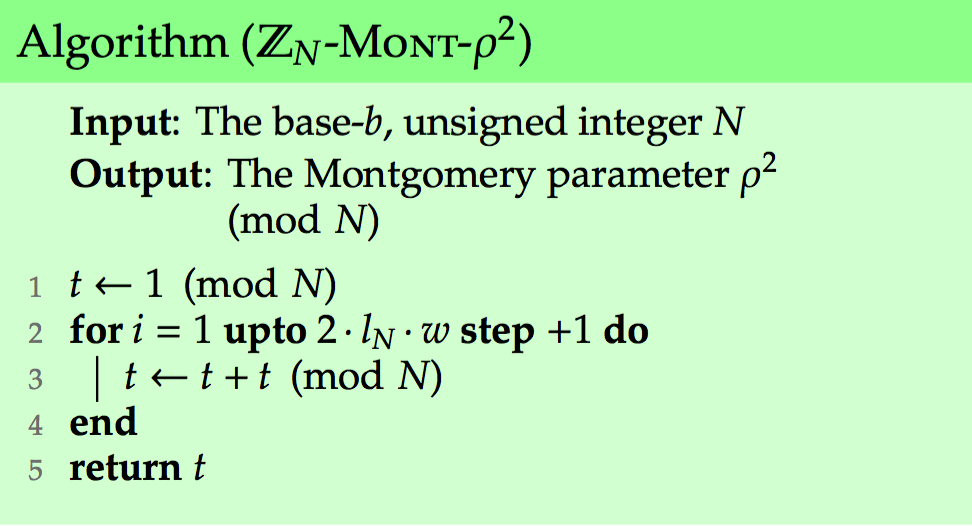03-072055

#### 蒙哥马利算法理解12-043264

#### Montgomery模乘算法

08-287821

#### 加密算法基础- Montgomery(蒙哥马利)乘法介绍

12-032983

#### 算法——指数取模运算（蒙哥马利算法）©️2020 CSDN 皮肤主题: 大白 设计师: CSDN官方博客点击重新获取扫码支付1.余额是钱包充值的虚拟货币，按照1:1的比例进行支付金额的抵扣。
2.余额无法直接购买下载，可以购买VIP、C币套餐、付费专栏及课程。余额充值